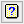1. Home
2. Microarray One Channel Regression Options

# Microarray One Channel Regression Options

Use this to set options for the One Channel Regression menu.## Display

The results to be displayed in the Output window:

 Model The regression model to be fitted. Summary The table giving a summary of the proportion of results that were significant at various levels of significance.

## Estimate constant term

Whether to estimate a constant as part of the regression model.

## Assign all possible parameters to factors

Whether to assign all possible parameters to factors and interactions.

## Pool information on each term

Whether to pool the information on each term in the analysis of variance. If you pool, for example with A + B, then you will get a single sums of squares for A + B, whereas if you don’t pool, then you get separate sums of squares for A and then B (after fitting A).

## Factorial limit on terms

Controls the level of interactions to be fitted. The value entered indicates the level of interaction allowed in the model (that is, set a limit on the maximum number of factors in the interaction terms that are fitted). For example, setting this to 1 will fit only the main effects (1 factor only in fitted terms), and setting this to 2 will only allow first order interactions of the form A.B, and higher order interactions such as A.B.C will not be included in the model. The terms not included in the model will be assigned to the error term.

## Type of residuals

Type of residuals to form (Deviance, Pearson, Simple). Deviance residuals (the default) are standardized by their estimated variance. The alternative Pearson residuals are defined in exactly the same way if the distribution is Normal, but for regression models with distributions other than Normal the two kinds of residual are different. Simple residuals are not standardized.

## Available data

This lists data structures appropriate for the field which currently has focus. You can double-click a name to enter it in the currently selected field or type the name.

## Weights

A variate specifying the weights for each of the units in the regression. If the field is left blank then equal weights of 1 will be used.

## Offset

Variate holding values to be used as an offset on the linear predictor scale. An offset is used to take account of a fixed contribution to the linear effects for each unit. If no offset is required then this field should be left blank.

## Action buttons

 OK Save the options settings and close the dialog. Cancel Close the dialog without making any changes. Defaults Reset the options to their default settings.

## Action IconsClear Clear all fields and list boxes.Help Open the Help topic for this dialog.